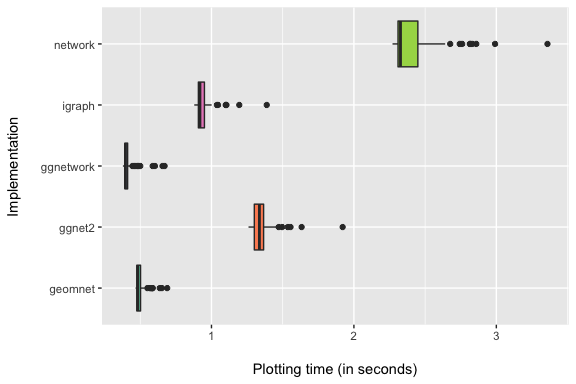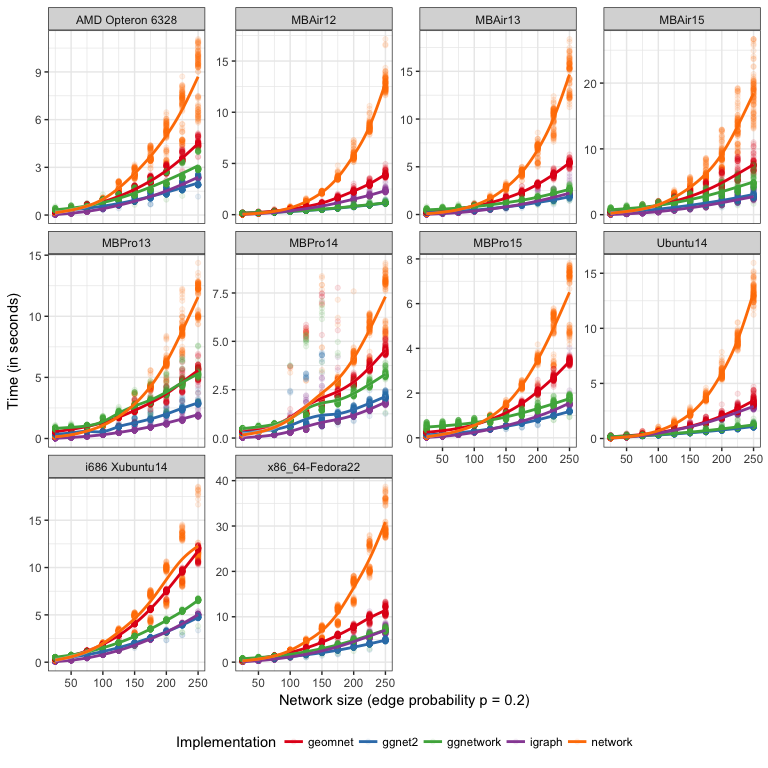# Introduction

This vignette provides access to the online supplement to the paper Network Visualization with ggplot2.

This vignette shows all of the code and gives access to the data for all of the examples and to reproduce all of the figures.

A summary of the speed comparisons is shown in the paper. A more detailed outline together with the code to reproduce findings is given in the next section.

# Speed Comparisons

This vignette compares the speeds of our package to the other ggplot2-based network visualization package ggnetwork, the GGally function ggnet2(), and the visualization capabilities of the igraph and network packages.

## Speed Comparison 1

In this first example, we visualize the protein-protein interaction network in the yeast species S. cerevisiae using several different methods.

First, load all of the necessary packages and data sets.

## igraph (current: v1.0.1)
#if (!require(igraph, quietly = TRUE)) {
#  install.package("igraph")
#}
library(igraph)

## network (current: v1.13.0)
#if (!require(network, quietly = TRUE)) {
#  install.package("network")
#}
library(network)

## ggnet2
#if (!require(GGally, quietly = TRUE)) {
#  install.packages("GGally")
#}
library(GGally)

## geom_net
library(geomnet) # also currently requires dplyr

## ggnetwork
#if (!require(ggnetwork, quietly = TRUE)) {
#  install.packages("ggnetwork")
#}
library(ggnetwork)

library(sna)
## ggplot2
library(ggplot2)

## other packages
library(dplyr)
library(tidyr)

data(protein, package = "geomnet")

Next, generate the network visualization of this network 100 times using the random layout option in each package and store the time to plot each run.

d = data.frame()

if (!file.exists("runtimes-protein-100.csv")) {

for (i in 1:100) {

cat("Iteration", sprintf("%3.0f", i), "/ 100\n")

n = as.matrix(protein$edges[, 1:2]) n = igraph::graph_from_edgelist(n, directed = FALSE) t1 = system.time({ plot(n, vertex.label = NA, layout = layout_randomly) }) n = network(protein$edges[, 1:2], directed = FALSE)

t2 = system.time({
plot(n, coord = gplot.layout.random(n, NULL))
})

t3 = system.time({
print(ggnet2(n, mode = "random"))
})

t4 = system.time({
print(ggplot(data = protein$edges, aes(from_id = from, to_id = to)) + geom_net(layout = "random")) }) t5 = system.time({ print(ggplot(ggnetwork(n, layout = "random"), aes(x, y, xend = xend, yend = yend)) + geom_edges() + geom_nodes()) }) d = rbind(d, data.frame( iteration = i, igraph = t1, network = t2, ggnet2 = t3, geomnet = t4, ggnetwork = t5, row.names = NULL )) } write.csv(d, file = "runtimes-protein-100.csv", row.names = FALSE) } Finally, compare the 100 runtimes for each method side-by-side. In the resulting plot below, we use our own data, which we have made part of the ggCompNet package in form of the data object runtimes_protein. data(runtimes_protein, package = "ggCompNet") g = runtimes_protein %>% gather(Visualization approach, time, -iteration) ggplot(g, aes(x = Visualization approach, y = time, fill = Visualization approach)) + geom_boxplot() + scale_fill_brewer(palette = "Set2") + coord_flip() + labs(y = "\nPlotting time (in seconds)", x = "Implementation\n") + guides(fill = FALSE)# Speed Comparison 2 The figure summarizes the results of these benchmarks using a convenience sample of machines accessible to the authors, including authors’ hardware and additional results from friends’ and colleagues’ machines. The data containing runtimes of for all of these machines can be found here. This time, we visualize graphs of various sizes that we generated randomly with edge probability 0.2 using the sna package. The sizes we generated are overall fairly small, from 25 to 250 nodes, going up by 25 each time. Each network visualization method was test 100 times in total for each size of network. # note: plot.network might be slower because it actually prints the plots, so to # compare the methods, we need to evaluate the ggplot plots by printing them too # create 10 files per size for (k in 0:9) { # the 10 different sizes for (i in seq(250, 25, -25)) { f = paste0("runtimes-", sprintf("%04.0f", i), "-", k, ".csv") if (!file.exists(f)) { d = data.frame() # in each file store the runtimes of plotting 10 random graphs # do this for each of the 5 methods for (j in 1:10) { r = sna::rgraph(i, tprob = 0.2) cat("Network size", i, "iteration", sprintf("%3.0f", 10 * k + j), "/ 100\n") n = igraph::graph_from_adjacency_matrix(r, mode = "undirected") t1 = system.time({ plot(n, vertex.label = NA) }) n = network::network(r, directed = FALSE) t2 = system.time({ plot.network(n) }) t3 = system.time({ print(ggnet2(n)) }) e = data.frame(sna::as.edgelist.sna(n)) t4 = system.time({ print(ggplot(data = e) + geom_net(aes(from_id = X1, to_id = X2))) }) t5 = system.time({ print(ggplot(ggnetwork(n), aes(x, y, xend = xend, yend = yend)) + geom_edges() + geom_nodes()) }) d = rbind(d, data.frame( network_size = i, iteration = 10 * k + j, igraph = t1, network = t2, ggnet2 = t3, geomnet = t4, ggnetwork = t5, row.names = NULL )) dev.off() } write.csv(d, f, row.names = FALSE) } } Again, for this plot we use our data that is available on Github. Network sizes are plotted horizontally, execution times of 100 runs under each visualization approach are plotted on the $$y$$-axis. Each panel shows a different machine as indicated by the facet label. Note that each panel is scaled separately to account for differences in the overall speed of these machines. # get all files containing runtime information and combine them into a single data frame. g = list.files(pattern = "runtimes-(.*)csv$") %>%
gather(Visualization approach, time, -network_size, -iteration) %>%
group_by(network_size, Visualization approach) %>%
q95 = quantile(time, 0.95))
## geom_smooth() using method = 'gam'The plotting routine in the network package is by far the slowest across all machines, while the plotting with igraph is generally among the fastest. Our three approaches generally feature in between these two, with ggnet2 being as fast or faster than igraph plotting, followed by ggnetwork and geomnet, which is generally the slowest among the three. These differences become more pronounced as the size of the network increases.